# How many parameters are there for an hMM model?

Let us calculate the number of parameters for bi-gram hMM given asLet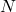be the total number of statesandbe the vocabulary size andbe the length of the sequence

1. Before directly estimating the number of parameters, let us first try to see what are the different probabilities or rather probability matrix we have.
2. Once we know the probability matrix, we can estimate the parameters by its size.
3. If you’re thinking how does a probability matrix appear, notice that we have conditional probabilities here,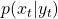and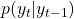or probability expression involving 2 variables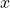andsuch as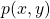, hence we should have the probability matrix.
4. So forwe have a probability matrix where each rowis a state and each column is an output variable. Hence this matrix is of size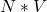leading to these many parameters.
5. Similarly for, we havexmatrix and hence same number of parameters.
6. From (d) and (e), we have at least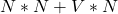parameters.
7. Now think carefully if we there is anything which we missed in our calculation.
8. We also have start tokens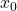and initial state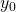. In State Probability matrix explained in (e), we have one more row but columns are still N. Therefore number of parameters due to initial statebecomes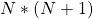9. Once you’re convinced, you’ll get to know that total number of parameters in the above hMM model are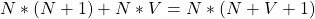10. Give a try for a generalfor n-gram hMM!